NCERT Questions

Class 8
Chapter 16 Class 8 - Light

## Two mirrors meet at right angles. A ray of light is incident on one at an angle of 30° as shown in Fig. 16.19. Draw the reflected ray from the second mirror.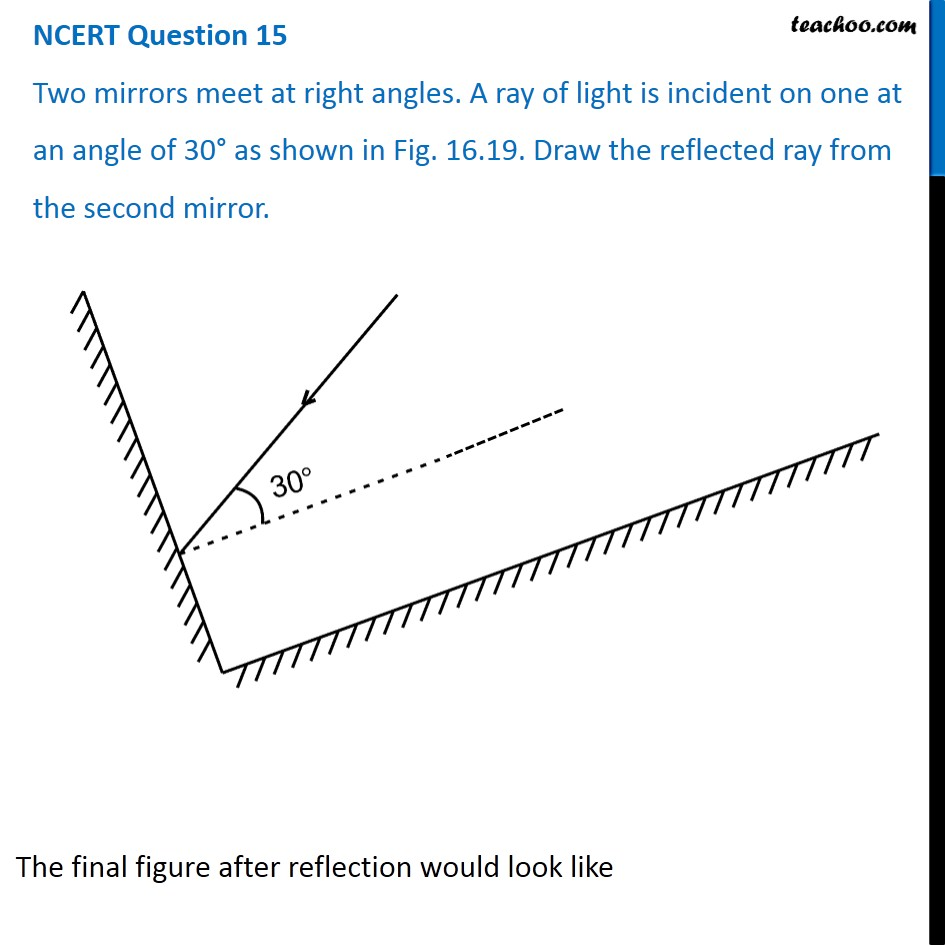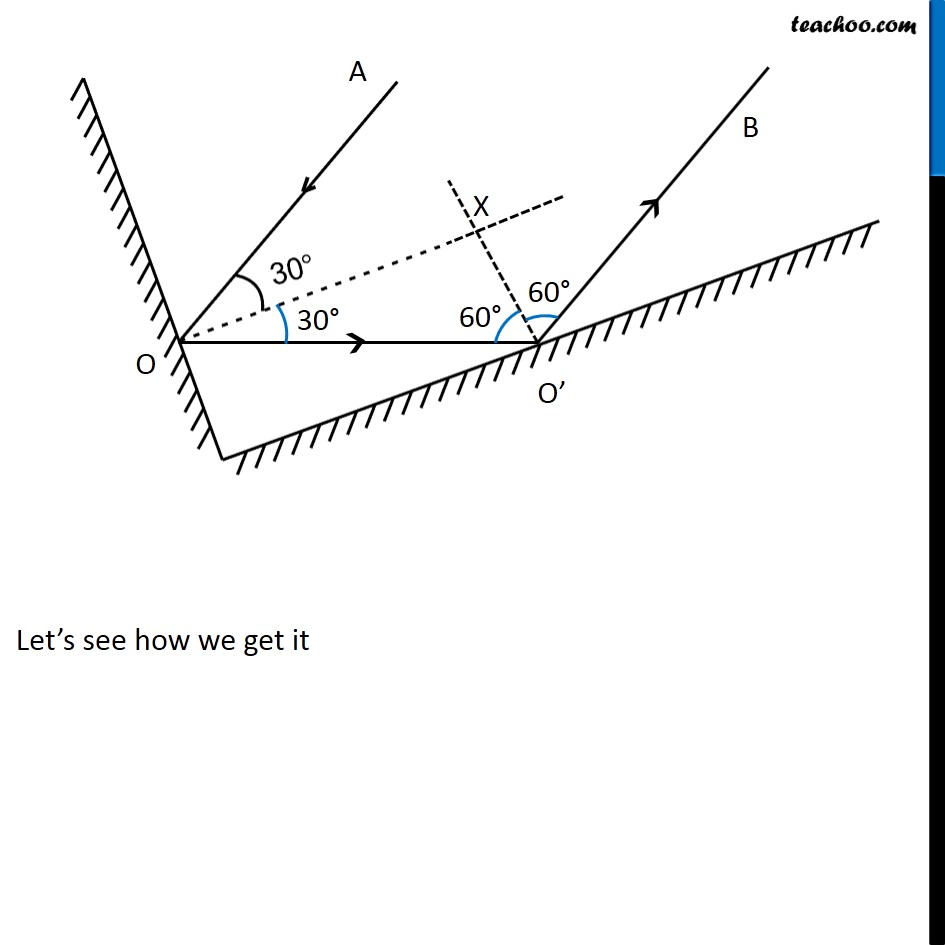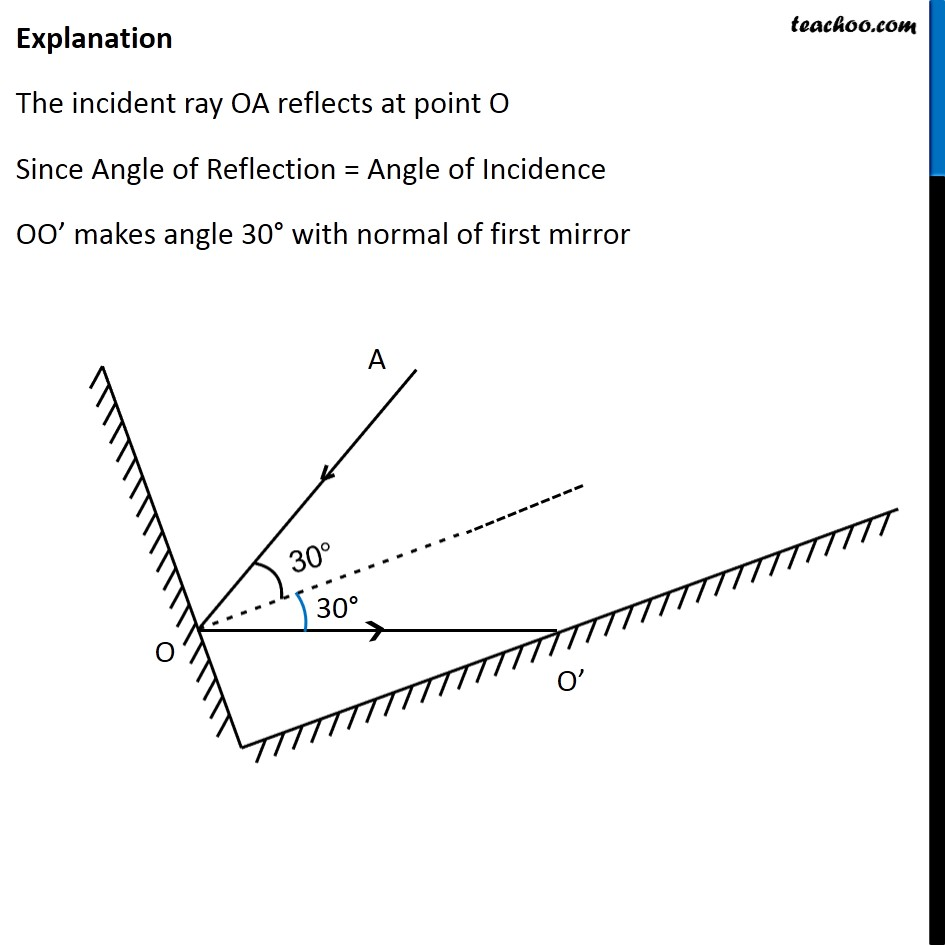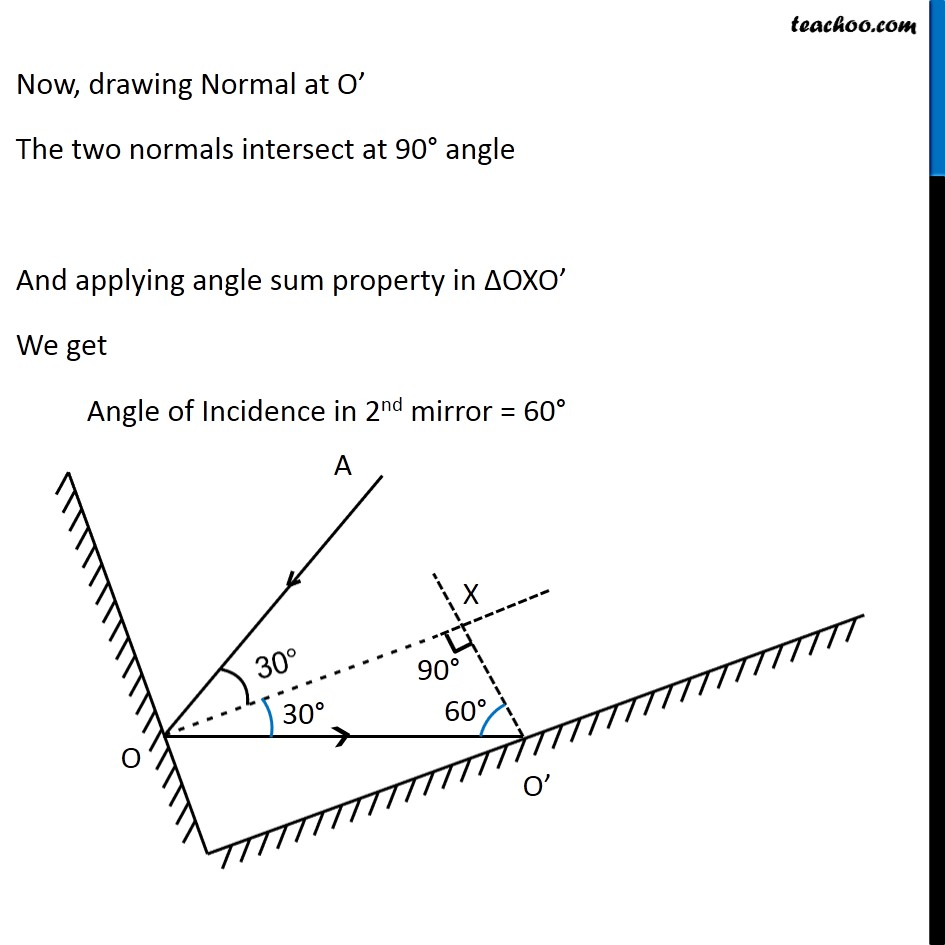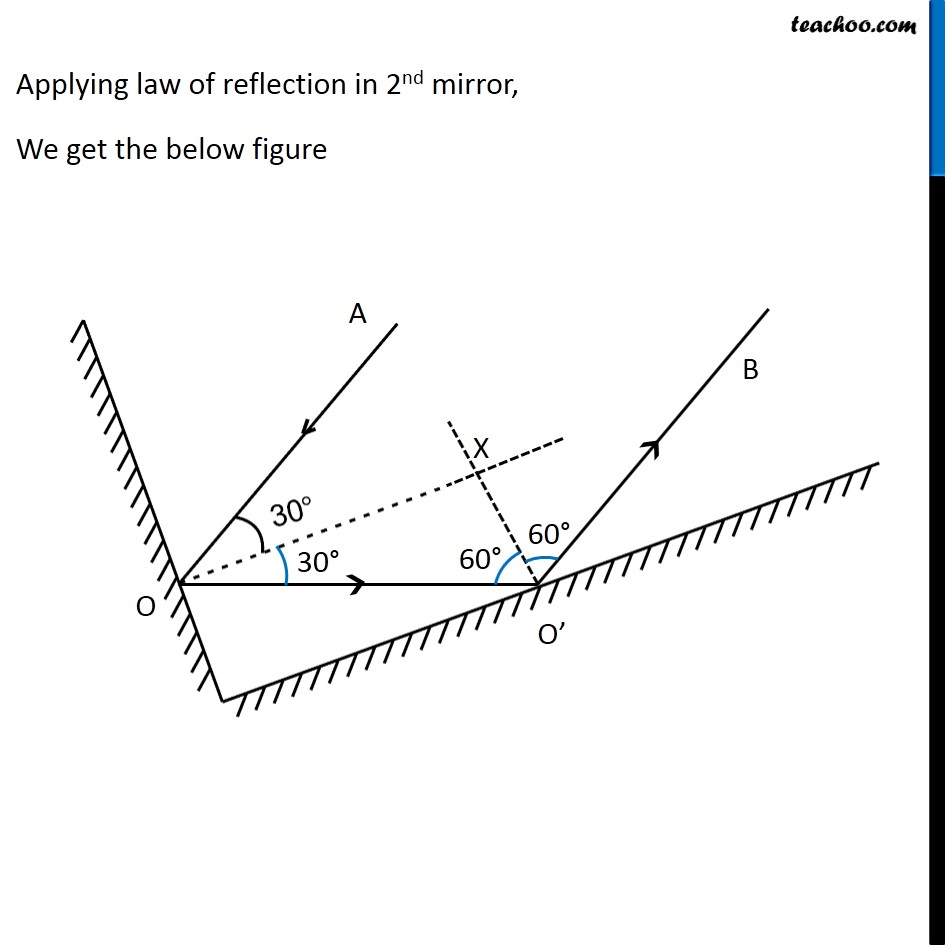Get live Maths 1-on-1 Classs - Class 6 to 12

### Transcript

The final figure after reflection would look like Let’s see how we get it Explanation The incident ray OA reflects at point O Since Angle of Reflection = Angle of Incidence OO’ makes angle 30° with normal of first mirror Now, drawing Normal at O’ The two normals intersect at 90° angle And applying angle sum property in ΔOXO’ We get Angle of Incidence in 2nd mirror = 60° Applying law of reflection in 2nd mirror, We get the below figure